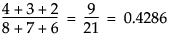Quality and Process Methods > Attribute Gauge Charts > Statistical Details for Attribute Gauge Charts
Publication date: 11/29/2021

# Statistical Details for Attribute Gauge Charts

For the first chart in Figure 6.5 that plots all X, Grouping variables on the x-axis, the percent Agreement is calculated as follows: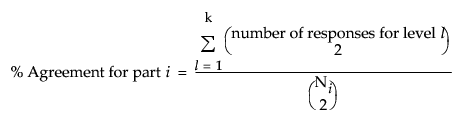For the second chart in Figure 6.5 that plots all Y, Response variables on the x-axis, the percent Agreement is calculated as follows: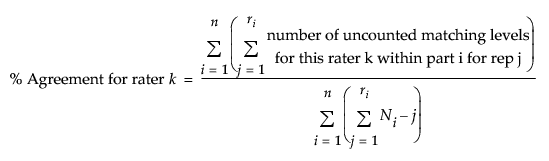Note the following:

n = number of parts (grouping variables)

ri = number of reps for part i (i = 1,...,n)

m = number of raters

k = number of levels

Ni = m x ri. Number of ratings on part i (i = 1,...,n). This includes responses for all raters, and repeat ratings on a part. For example, if part i is measured 3 times by each of 3 raters, then Ni is 3 x 3 = 9.

For example, consider the following table of data for three raters, each having three replicates for one part.

Table 6.1 Three Replicates for Raters A, B, and C

A

B

C

1

1

1

1

2

1

1

0

3

0

0

0

Using this table, you can make these calculations:

% Agreement =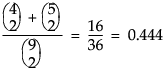% Agreement [rater A] = percent Agreement [rater B] =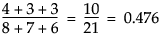and

% Agreement [rater C] =